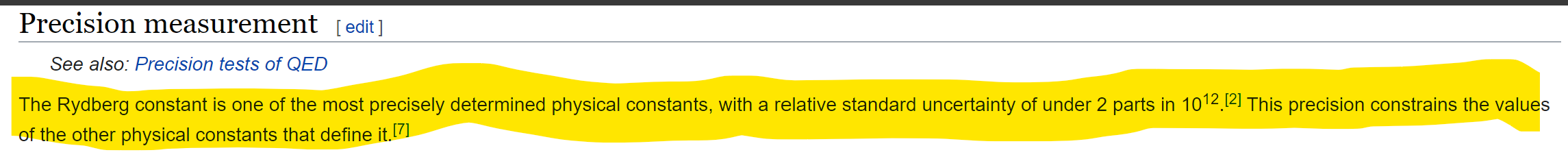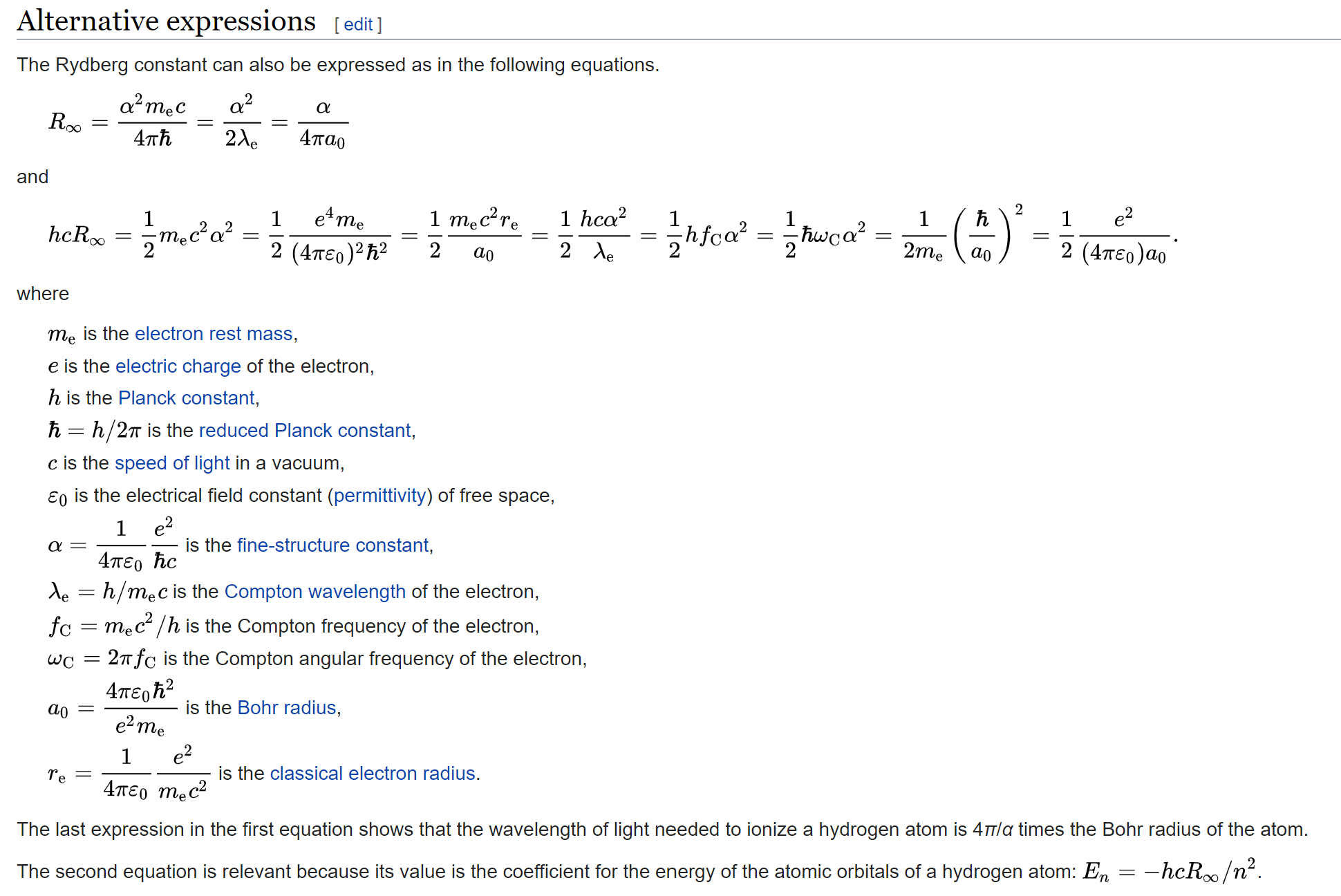## Wednesday, July 1, 2020

### Convergence: Harmonic Convergence

The post: visual-basic-in-excel-phi-ratio... demonstrates convergence.  For stable systems that demonstrate and define the coefficients of interaction, a periodic solution, $2\pi n$, periodic integer multiples of $2\pi$, clearly holds for the fundamental and higher order harmonics/orbits/states, thus the adjective "Harmonic" accompanies the word convergence... Harmonic Convergence.

So, an equation like $x^2-x-1=0$, via algorithm, convergences precisely on phi, ${1+\sqrt5}\over2$.  What about higher order polynomials, do they also converge?  Some do, some don't.  (The Math Oracle has spoken)

The Rydberg equation does not converge, however, the full Rydberg, that includes the $m_e\over m_p$ electron to proton mass ratio term, DOES converge.

DOES converge* when you use the correct equation for the mass ratio involving the proton radius.  Since it is a quantized solution, and harmonic (the hydrogen atom is being modeled like a 2 dimensional vibration of a string, but it's the atom and its quantum energy states.

The Harmonic Reactor
more later...

* I also suspect it converges (but maybe not) just using the electron and proton mass, however, need to check.  If so, it means one did not have to wait for the radius equation. if...

The Surfer, OM-IV

## Sunday, June 28, 2020

### He's Playing G-d With the Constants! Son of ahhhh...Out of the Aether Triumph Pepe appears
But the Rydberg constant is their most sacred and proud constant:close...

more later

The guys over at NIST are like: